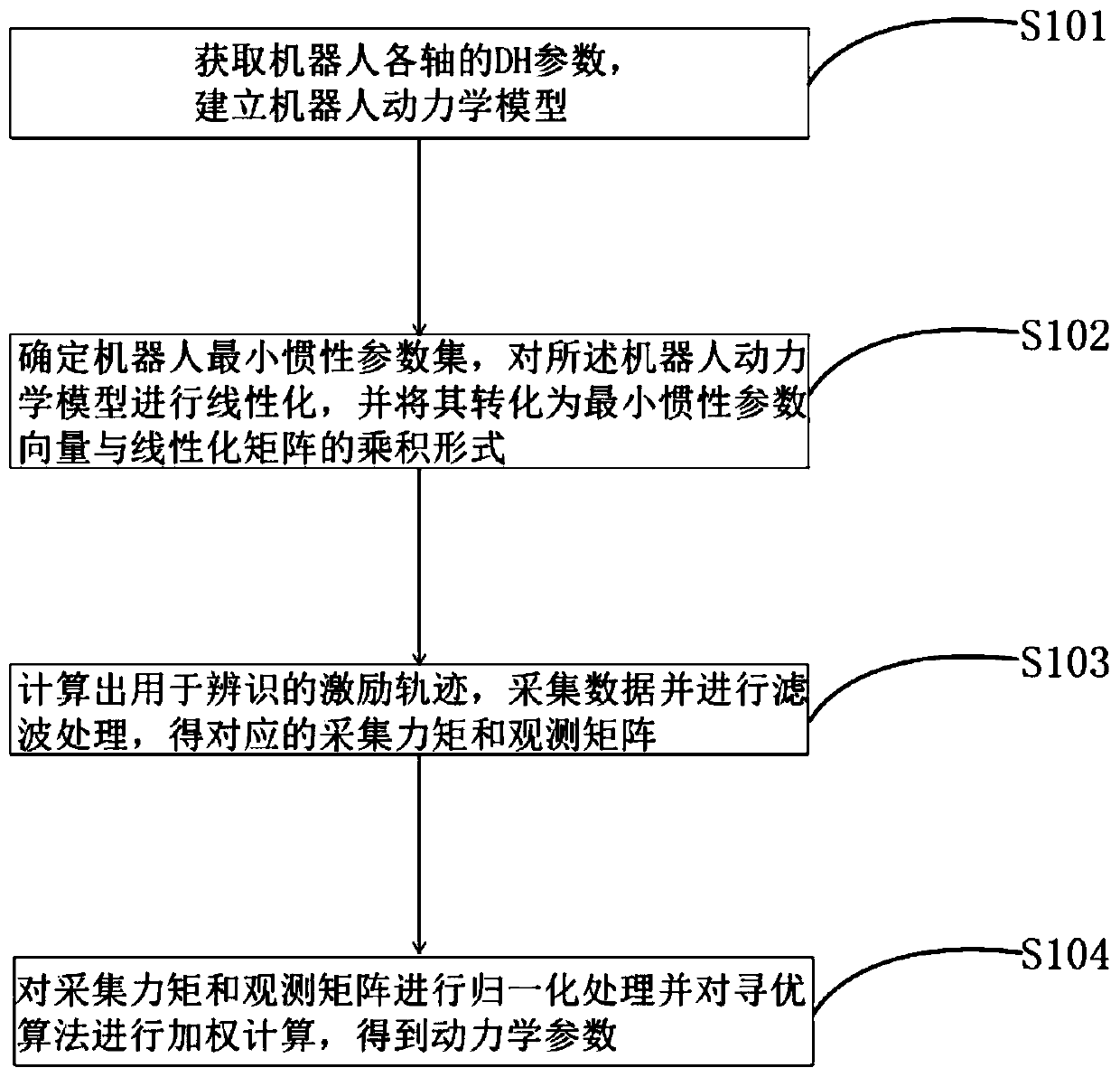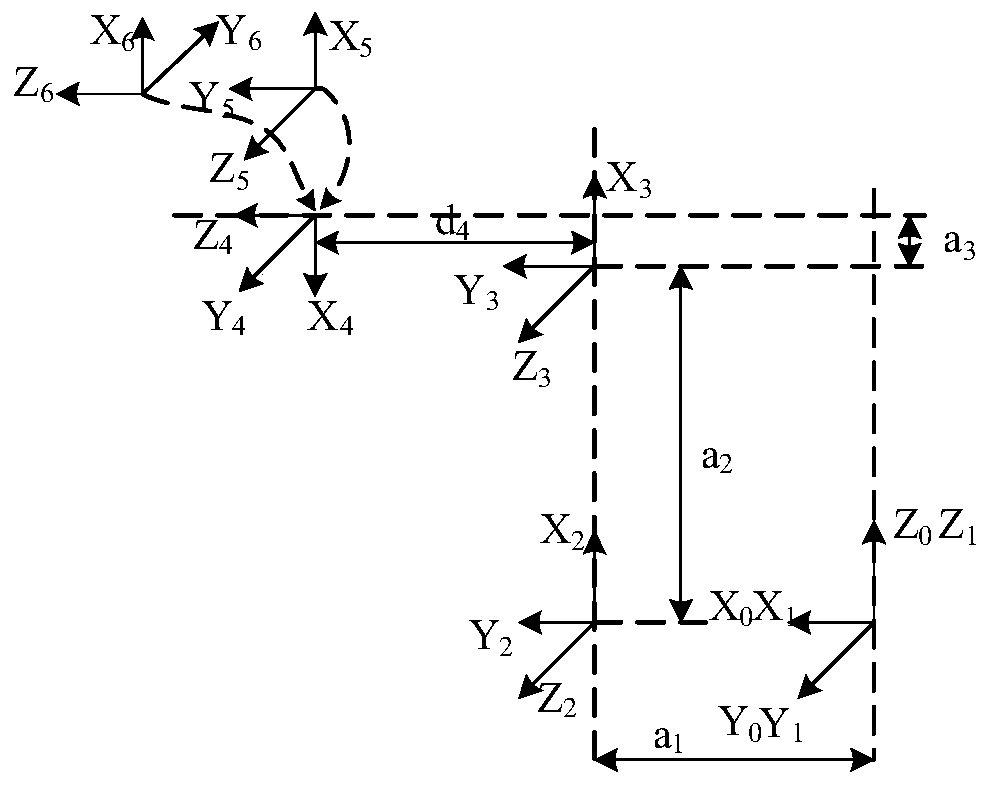# Overall kinetic parameter identification method based on series robot

## A technology of dynamic parameters and identification methods, applied in the field of overall dynamic parameter identification based on serial robots, can solve the problems of excessive axis parameter identification accuracy errors and defects in collected data, and achieve the effect of improving reliability.

Pending Publication Date: 2020-08-07
WUXI XINJIE ELECTRICAL
11 Cites 3 Cited by

## AI-Extracted Technical Summary

### Problems solved by technology

 The purpose of the present invention is to overcome the above-mentioned problems in the prior art, and propose a series identification scheme based on Markov chain and weighting thought, which can solve many problems existing in the identification of overall parameters, such as the overall Or the problem of t...
View more

## Abstract

The invention discloses an overall kinetic parameter identification method based on a series robot, and belongs to the technical field of robot control. The overall kinetic parameter identification method comprises the steps: DH parameters of all axes of the robot are obtained, and a robot kinetic model is established; a minimum inertia parameter set of the robot is determined, and the robot kinetic model is linearized and converted into a form of a product of a minimum inertia parameter vector and a linearized matrix; an excitation track used for identification is calculated, data are collected and filtered, and a corresponding collecting moment and measurement matrix are obtained; and the collecting moment and the measurement matrix are normalized, weighted calculation is conducted on anoptimization algorithm, and kinetic parameters are obtained. According to the overall kinetic parameter identification method based on the series robot, various problems, such as the problem, causedby inconsistency of series axis scales, of excessive error of identification precision of overall or certain axis parameters, the problem of collecting data imperfection and the problem of data credibility, in overall parameter identification can be solved; and meanwhile the kinetic parameters of the robot can be identified on line, and the reliability of parameter identification can be greatly improved.

Application Domain

Technology Topic

Robot controlAlgorithm +4

## Image

•••## Examples

• Experimental program(3)

### Example Embodiment

 Example 1
 In the case of off-line parameter identification, an overall dynamic parameter identification method based on tandem robots includes the following steps:
 Step (1): Obtain the DH parameters of each axis of the robot, and establish the conversion relationship matrix between the linkage coordinate system and each axis. The Newton-Euler iterative method is used to establish the overall dynamics model of the tandem robot; the DH parameters include the rod length, rod torsion angle, joint distance and joint rotation angle of each axis of the robot;
 Step (2): Use robot geometric parameters to directly determine the minimum inertia parameter of the robot, linearize the robot dynamics model, and convert it into the product of the minimum inertia parameter vector and the linearization matrix;
 Step (3): Taking the condition number of the observation matrix as the optimization criterion, and taking the angle, angular velocity, and angular acceleration of each joint of the robot as the constraint conditions, an intelligent algorithm is used to design an excitation trajectory for identification, and relevant data are collected and filtered respectively. Obtain the corresponding acquisition torque and observation matrix; the filtering processing includes low-pass filtering, mean filtering, Kalman filtering, etc.;
 Step (4): Multiply the corresponding rows of the acquisition moment and the observation matrix in step (3) by the spatial weighting processing of the weights to obtain dynamic parameters.

### Example Embodiment

 Example 2
 In the case of online parameter identification, an identification method based on the overall dynamics parameters of a series robot includes the following steps:
 Step (1): Obtain the DH parameters of each axis of the robot, and establish the conversion relationship matrix between the linkage coordinate system and each axis. The Newton-Euler iterative method is used to establish the overall dynamics model of the tandem robot; the DH parameters include the rod length, rod torsion angle, joint distance and joint rotation angle of each axis of the robot;
 Step (2): Use robot geometric parameters to directly determine the minimum inertia parameter of the robot, linearize the robot dynamics model, and convert it into the product of the minimum inertia parameter vector and the linearization matrix;
 Step (3): Taking the condition number of the observation matrix as the optimization criterion, and taking the angle, angular velocity, and angular acceleration of each joint of the robot as the constraint conditions, an intelligent algorithm is used to design an excitation trajectory for identification, and relevant data are collected and filtered respectively. Obtain the corresponding acquisition torque and observation matrix; the filtering processing includes low-pass filtering, mean filtering, Kalman filtering, etc.;
 Step (4): The corresponding rows of the acquisition torque and the observation matrix in step (3) are respectively multiplied by the weighted spatial weighting processing, and the acquisition torque and the observation matrix collected at different time points are processed for time Weighting processing to obtain dynamic parameters; the time weighting processing is specifically: taking different matrix blocks of trajectory segment data for the data collected at different times, and forming all the matrix blocks into a Markov chain in descending order of the collection time, Multiply the weighted geometric sequence of the data of different matrix blocks to perform time weighting processing.

### Example Embodiment

 Example 3
 Take the six-axis serial robot body as an example to describe the scheme of robot dynamic parameter identification.
 Firstly, the coordinate system of the tandem robot rods is established, and the conversion matrix between each rod is established according to the DH parameters of the rods. DH parameters include rod length a i , The torsion angle of the member α i , Joint distance d i , Joint rotation angle θ i , Where i represents the joint number.
 Such as figure 2 with 3 As shown, this embodiment uses modified DH parameters, which are different from standard DH parameters. The rod coordinate system established by the modified DH parameters is the drive shaft coordinate system, and the conversion matrix between the coordinate systems can be calculated from the DH parameters, as (1) Shown:

 among them Is the conversion matrix between the i-1 and i-th coordinate system, cθ i =cos(θ i ),sθ i =sin(θ i ),cα i-1 =cos(α i-1 ),sα i-1 =sin(α i-1 ). The transformation matrix determines the transformation relationship between the position and posture of each joint.
 Commonly used methods for establishing robot dynamics are Newton-Euler method and Lagrangian method. Here, Newton-Euler method is used. The principle is the recursive solution of joint pose from inside to outside and joint torque from outside to inside. Recursive solution. Obtain the robot dynamics model as shown in formula (2):

 Where H is the inertia matrix, C is the Coriolis force and centripetal force solution matrix, G is the gravity vector, τ is the moment vector of each joint, q, Is the joint angle, angular velocity, and angular acceleration vector.
 The geometric parameters of the robot are used to directly determine the minimum inertia parameters of the robot and the number is 36 (H. Kawasaki and K. Kanzaki, 1991). Because the 36 minimum inertial parameters are linear combinations of 60 inertial parameters of 6 axes, and the dynamic equations are known as linear functions of 60 inertial parameters from the dynamic formula, they can be linearized.
 Use MATLAB for symbolic calculation to linearize the kinetic model announcement (2). The specific method is:
 1. Extract 10 inertia parameters of each axis (member mass m, moments of inertia Ixx, Ixy, Ixz, Iyy, Iyz, Izz, center of gravity Mx, My, Mz) to form an inertia parameter vector of 60 parameters;
 2. After separating 60 parameters from the kinetic equations, an observation matrix with 6 rows and 36 columns is formed;
 3. According to the combination relationship between the original 60 inertial parameters and 36 minimum inertial parameters, perform the corresponding elementary matrix transformation on the observation matrix with 6 rows and 36 columns in 2 and delete the extra rows to obtain an observation matrix with 6 rows and 36 columns. . The linearized formula is:

 Where Φ is an observation matrix with 6 rows and 36 columns, and θ is the minimum inertial parameter vector of the 36-dimensional robot.
 Design the first excitation trajectory with Fourier curve, that is, the angle-time function of each joint is:

 Where q 0 Is the initial position, ω f Is the frequency, a k , B k (k=1, 2, 3...N) are coefficients.
 Taking N=5 as an example, q 0 When certain conditions are met, the angles of the initial point and the end point of q can be kept at 0. Given frequency ω f , The observation matrix Φ is a k , B k The function.

## PUM## Description & Claims & Application Information

We can also present the details of the Description, Claims and Application information to help users get a comprehensive understanding of the technical details of the patent, such as background art, summary of invention, brief description of drawings, description of embodiments, and other original content. On the other hand, users can also determine the specific scope of protection of the technology through the list of claims; as well as understand the changes in the life cycle of the technology with the presentation of the patent timeline. Login to view more.

## Housing for an optical reader

ActiveUS7147162B2improve reliability
Owner:HAND HELD PRODS

## Installation management method, server and terminal for application program

Owner:LENOVO (BEIJING) CO LTD

## Unmanned aerial vehicle flight control system having multisensor redundant backup

ActiveCN105867414AIncrease flight control weight and costImprove reliability
Owner:ZHEJIANG UNIV

## Method for driving semiconductor device

Owner:SEMICON ENERGY LAB CO LTD

## File upgrading method and terminal device

InactiveCN101557308AImprove reliability
Owner:HUAWEI DIGITAL TECH (CHENGDU) CO LTD

## Classification and recommendation of technical efficacy words

• Improve reliability

## Method and system for reducing thermal pole tip protrusion

InactiveUS6909578B1improve reliability
Owner:WESTERN DIGITAL TECH INC

## Multiple branch predictions

ActiveUS20050268075A1improve reliabilityfacilitate availability
Owner:ORACLE INT CORP

## Integrated circuit device and method of producing the same

InactiveUS7084507B2good resistanceimprove reliability
Owner:FUJITSU LTD

## Multifunction smoke alarm unit

ActiveUS20100137928A1good flexibility , fatigue resistance and flex lifeimprove reliability
Owner:WL GORE & ASSOC INC
Who we serve
• R&D Engineer
• R&D Manager
• IP Professional
Why Eureka